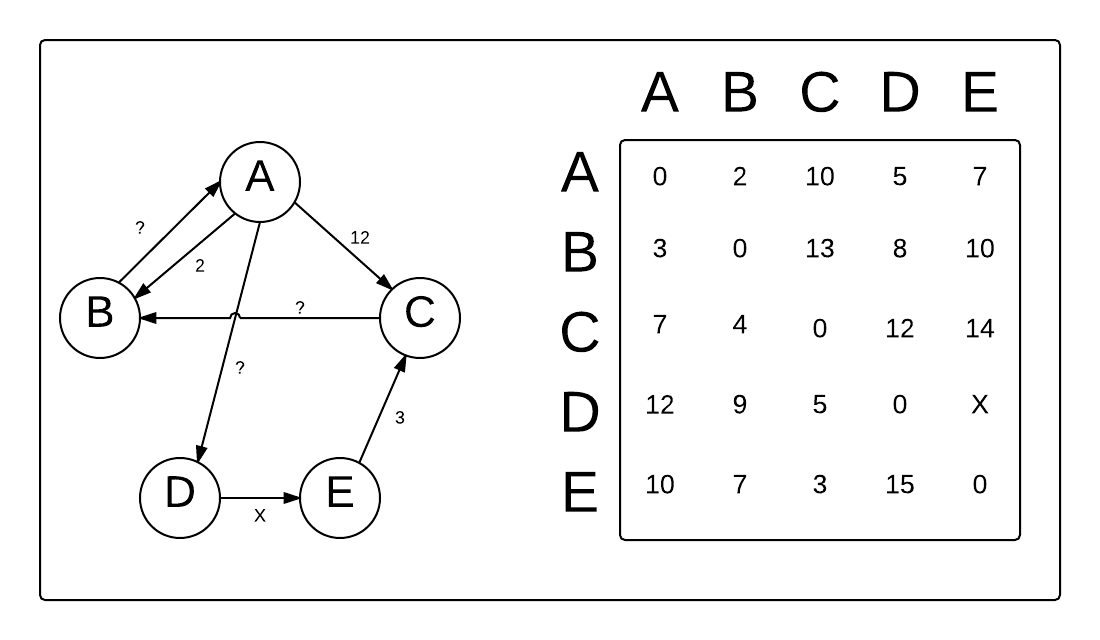# Solve for X in the graph and matrix below

The following picture shows a graph, $G$, with vertices $V = {A, B, C, D, E}$ with edge set $E$. Some edge weights are shown, and others are not.

Also below is the resulting matrix $D$ from the Floyd-Warshall algorithm. In this matrix, $D[i][j]$ shows the distance between vertex $i$ and vertex $j$ in the graph. Solve for $X$.Graph and distance matrix

×